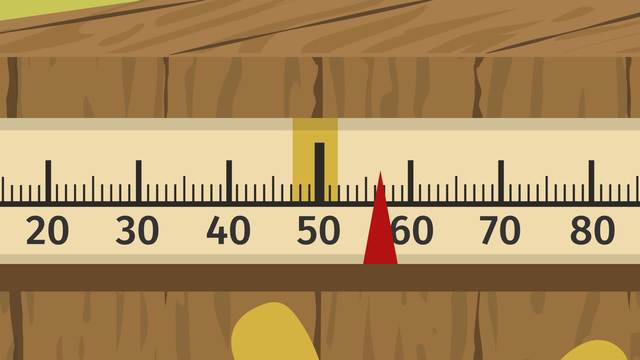# Percent ErrorRate this video

Ø 5.0 / 2 ratings

The authorEugene Lee

## Information about the videoPercent Error

After this lesson, you will be able to compute absolute error and solve percent error problems.

The lesson begins by defining absolute error and illustrating how it is calculated. This illustration leads to a method for using absolute error to find the percent error.

Learn about percent error by helping Harriet figure out what went wrong with her new Plunk ‘N Dunk tank at the Otter this World amusement park!

This video includes key concepts, notation, and vocabulary such as: absolute error (the distance on a number line between the measured value and the exact value); measured value (a single actual value, result, or amount); exact value (the target or estimated value); percent error (how close the measured value is in relation to the exact value).

Before watching this video, you should already be familiar with absolute value and solving problems using percentages.

After watching this video, you will be prepared to learn how to solve other types of multi-step percent problems.

Common Core Standard(s) in focus: 7.RP.A.3 A video intended for math students in the 7th grade Recommended for students who are 12-13 years old

### TranscriptPercent Error

At the tempered timber amusement park, Otter This World, Plunk 'N Dunk is the park's most popular attraction. Customers try to stop the needle within a 10% error of the exact value, 50 inches. If a customer is successful, dunk goes the weasel sittin' in the dunk tank. But, strange things have been happening at night. Lately, only a few customers have been able to stop the needle within the accepted percent error, so naturally, the weasels have been kicking back and relaxing in the dunk tank. Much to the chagrin of the weasels, Harriet, the park's meticulous manager, is investigating. In order to help Harriet figure out what went wrong, we'll need to understand percent error. To play Plunk 'N Dunk, customers try and stop a needle on the number 50, but if customers stop the needle within a 10 percent error of 50, or the "dunk zone", then dunk goes the weasel. Currently, the Dunk Zone has an exact value of 50 inches, and goes from the 47 inch mark on the left to the 52 inch mark on the right. To understand percent error, we first have to know about absolute error. Absolute error is the absolute value of the difference between the exact value and the measured value. In our case, the exact value is 50 inches and the measured value is 47 or 52 inches. Let's start with a measured value of 52 inches and find the absolute error. We first need to subtract the numbers, giving us the absolute value of 2. Since absolute value means to take the distance from zero on the number line, we're left with an absolute error of 2. Now we can find percent error with ease. Percent error is found by first dividing the absolute error by the absolute value of the exact value, then multiplying by 100 to get a percentage. We already know the absolute error, 2 inches, and we already know the exact value is 50 inches, so let's substitute that here. Dividing our absolute error by the absolute value of the exact value, 50, gives us 0.04. And when we multiply 0.04 by 100, we get a percent error of 4 percent! That's definitely not the 10% error that it should be. So let's look at the percent error to the 47 inch mark. We know that, to find percent error, we first need the absolute error divided by the absolute value of the exact value. Then, this is multiplied by 100, giving us our percent error. But how do we find absolute error, again? Remember, absolute error is how far our measured value is from the exact value we want. Therefore, we subtract the two numbers and take their absolute value because we want their distance apart and that's always positive. Now, we just need to substitute and solve! This time, our measured value is 47 inches and our exact value is still 50 inches. Subtracting 50 from 47 gives us negative 3. The absolute value of negative 3 is 3. That is our absolute error. Percent error takes it a bit further and shows us the error as a percentage of the exact value. Using substitution, we can take the absolute error, 3, and divide it by the absolute value of our exact value, 50, before multiplying by 100. This leaves us with 0.06, which, when multiplied by 100, gives us our percent error of 6%. That's also not the 10% that we want. Before contacting Harriet, let's review what we've learned. Absolute error is found by subtracting the exact value from the measured value and then taking the absolute value of the result. While percent error is found by dividing the absolute error by the absolute value of the exact value, then multiplying by 100 to get a percentage, percent error tells you how close a measured value is in relation to the exact value. In this example, the weasels decreased the allowed percentage error, which made it more difficult for the customers to stop the needle in the Dunk Zone. Now that we've helped Harriet understand percent error, she's come down to inspect the faulty attraction. She notices someone has changed the numbers on the box! Those weasels, they think they're so slick. Harriet's got just the surprise for them! There, that should fix it!